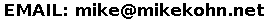CONTENTS

Home
Software
Electronics
Android / iOS
Music
Resume
Contact

GitHub

Posted: December 16, 2015

Introduction

Here's a small piece of electronic artwork: a half adder made out of transistors. This is really a pretty basic circuit, although as seen from the wires on the circuit below, kind of a pain in the neck to wire up. I left out pull-down resistors on the base pins of all the transistors which was probably not the best idea. If anyone is repeating this project, put 10k resistors from every transistor gate to ground and remove the resistors from the switches. This project ends up being an extension of an earlier XOR gate project I did with the difference being it wasn't done with conductive ink and there is also an AND gate added.

Below is the half adder circuit made from 2N7000 MOSFETs. The two LED's at the bottom left represent the answer to the math problem button 1 + button 2. The buttons are lined up above the 1's column and the LED on the left is the 2's column (can also be thought of as the carry bit).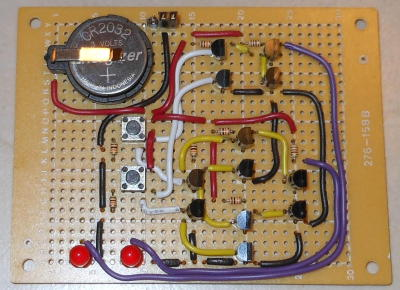So basically the a half adder is simply an XOR gate (the cluster of 8 transistors in a diamond formation at the lower part of the circuit) to calculate the value of addition between two 1 bit binary numbers. The carry bit is only set if both digits in the addition are 1's, so this is just simply an AND gate (the cluster of 4 transistors above the XOR gate).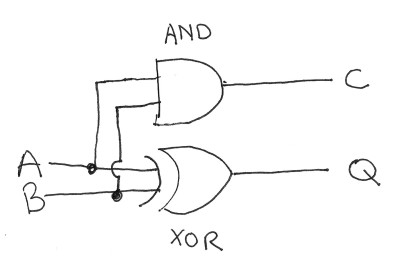Since all logic gates (NAND, AND, NOR, OR, XOR, NOT) can simply be made out of NAND gates, the adder can be broken up into a set of NAND gates:And since NAND gates are simply made out of two transistors, the adder can be broken down one more time into a set of transistors. In the circuit above I made each NAND gate out of 2 MOSFETs in the configuration below.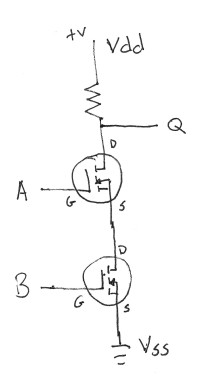NAND gates could also be made from bipolar NPN transistors: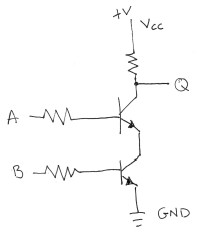Below, from left to right, two 2N7000 MOSFET transistors, two 2N7000's soldered together to make a NAND gate, and a 7400 IC quad NAND gate IC.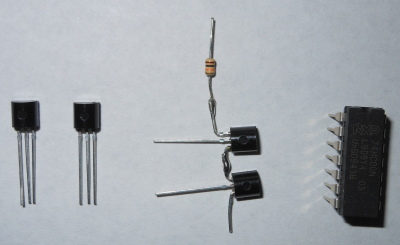To make the circuit into a full adder, a carry bit would be included into the equation. This would require 2 XOR gates for bit 0 and to calculate carry, the equation (A AND B) OR ((A XOR B) AND C) would be used.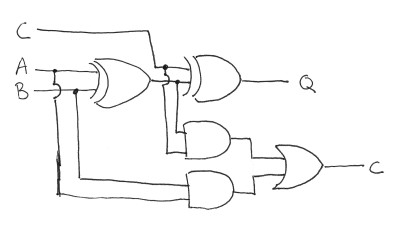Related Projects @mikekohn.net